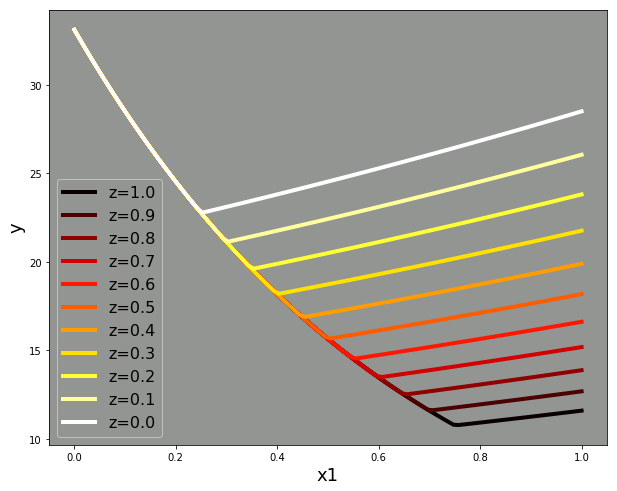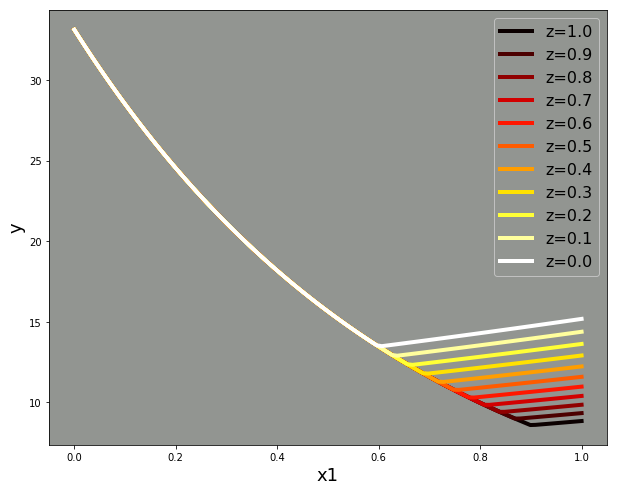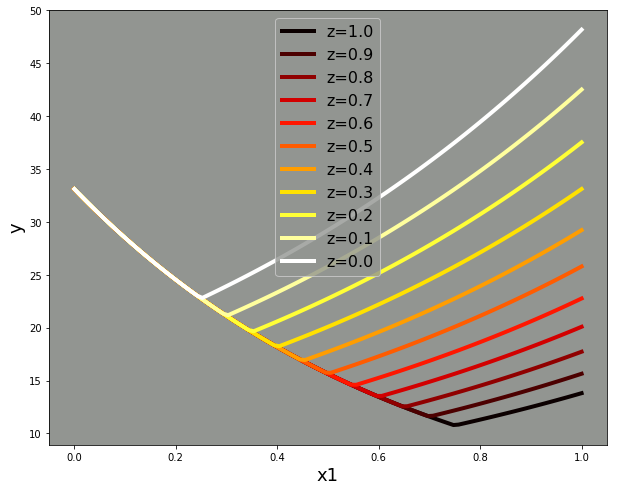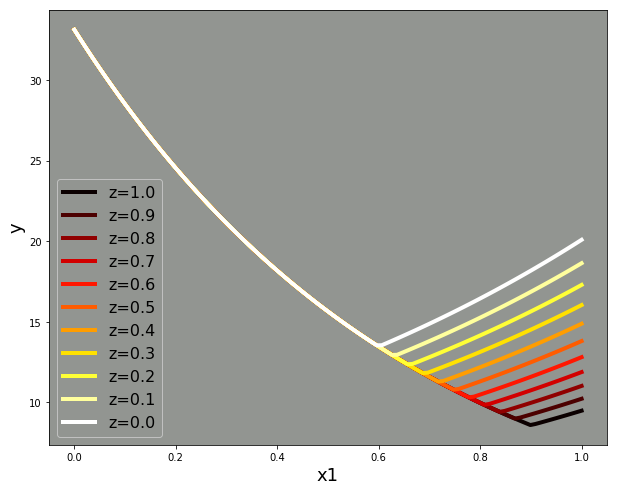In :
import sys
import numpy as np
import pandas as pd
import matplotlib.pyplot as plt
%matplotlib inline
import seaborn as sns

In :
# function to plot model predictions

def plotModel(p):
zs = np.linspace(0, 1, 11, endpoint=True)
plt.figure(figsize=(10,8))
plt.gca().set_facecolor('xkcd:gray')
plt.gca().set_prop_cycle('color',plt.cm.hot(np.linspace(0,1,len(zs))))

for z in zs[::-1]:
x1 = pd.Series(np.linspace(0, 1, 100, endpoint=True), name='x1')
z1 = pd.Series(z * np.ones(len(x1)), name='z1')
df = pd.concat([x1, z1], axis=1)
plt.plot(df['x1'], model_mean(p, df), label='z='+f'{z:.1f}', linewidth=4)

plt.xlabel('x1', fontsize=18)
plt.ylabel('y', fontsize=18)
plt.gca().legend(prop={'size': 16}).get_frame().set_facecolor('xkcd:gray')
plt.show()


## Broken Line Model Definition¶

In :
# Model Definition _________________________________________________________________________

def switch(params, data):
intercept = params
slope = params
switchpoint = intercept + (slope * data['z1'])
switchpoint = np.clip(switchpoint, 0, 1)
return switchpoint

def model_mean(params, data):
# params = [preIntercept, preSlope, slopeDiff, switchIntercept, switchSlope]

intercept = params
slope = params
slopeDiff = params
switchPoint = switch(params, data)
isPost = data['x1'] > switchPoint

mu = intercept + (slope * data['x1']) + (isPost * (slopeDiff * (data['x1']-switchPoint)))

return np.exp(mu)

def model_gen(params, data):
return scipy.stats.poisson.rvs(model_mean(params, data))

def model_like(params, data):
return - np.sum(np.log(scipy.stats.poisson.pmf(data['y'], model_mean(params, data))))


## Fixed switchpoint parameter values (taken from Muggeo, 2003)¶

params1 = [3.5, -1.5, 1.8, .5, 0]

params2 = [3.5, -1.5, 1.8, .75, 0]

params3 = [3.5, -1.5, 2.5, .5, 0]

params4 = [3.5, -1.5, 2.5, .75, 0]

## Proposed switchpoint parameter values¶

The objective here is to keep the Muggeo values as much as possible, but modified so as to permit parametric variation of the switchpoint (e.g., the post-switch slope should be non-zero). Recall that z1 is drawn from U(0,1), meaning that E(z1)=0.5. So a switchpoint intercept of .25 and a switchpoint slope of .5 would yield an average switchpoint of 0.25 + (.5 * .5) = .5 and would fall within the interval [0.25, .75]. To mirror the fixed-switchpoint parameter values listed above, I propose the following values:

params1 = [3.5, -1.5, 1.8, .25, .5]

params2 = [3.5, -1.5, 1.8, .6, .3]

params3 = [3.5, -1.5, 2.5, .25, .5]

params4 = [3.5, -1.5, 2.5, .6, .3]

In all cases, the mean switchpoint is equal to the (fixed) switchpoint aboved. In the first and third instances, the switchpoints fall within the interval [0.25, .75]. In the second and fourth, the switchpoints fall within the interval [0.6, 0.9]. This means that the range of the switchpoints in the former cases is 0.5 whereas the range in the latter is 0.3 and in all cases the range of switchpoints is well confined within the range of x1 (i.e., [0, 1]). Given that we have decided to investigate cases in which the switchpoints fall outside the measured range as one of our "extensions", keeping the current simulations "well behaved" seemed desirable.

Assuming none of that offends, let's take a look at what all this looks like!

In :
# Params1

params = [3.5, -1.5, 1.8, .25, .5]
plotModel(params)In :
# Params2

params = [3.5, -1.5, 1.8, .6, .3]

plotModel(params)In :
# Params3

params = [3.5, -1.5, 2.5, .25, .5]

plotModel(params)In :
# Params4

params = [3.5, -1.5, 2.5, .6, .3]

plotModel(params)